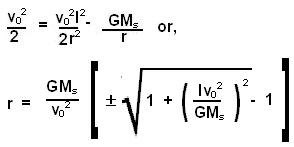## Tuesday, September 9, 2008

### Irodov Problem 1.210We shall solve this problem exactly as we solved 1.209, using conservation of energy and angular momentum.

The angular momentum of the planet when it was at infinity is given by mv0l and its total energy is only its kinetic energy since at infinity it has no potential energy. Suppose that at the planet's closest approach its distance from the sun is r and its velocity is v, the sun's mass is Ms and the planet mass is m, then we have,

Conservation of angular momentumConservation of energyFrom (1) and (2) we have,Now we can ignore the solution with negative sign since a negative value for r does not make any sense.